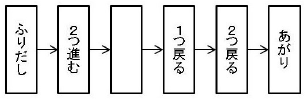Time Limit : sec, Memory Limit : KB

# Making Sugoroku

Taro made a backgammon game for a children's club event. To make the game more interesting, he wrote instructions on some of the squares of the backgammon except for " Start " and " Goal ", such as "Go forward 6" and "Go back 5". When the roulette wheel is turned, the player moves forward as many times as the number of squares that come up. If there is an instruction written on a stopped square, the player moves according to the instruction, but not according to the instructions on the square.

The roulette wheel is supposed to be able to produce any number between 1 and a certain number with equal probability. If the number is greater than the number that reaches the "Goal", or if following the instructions moves you ahead of the "Goal", you will move to the "Goal". On the other hand, if following the instructions would take you back before the "Start", then you go back to the "Start".However, depending on the instructions of the roulette and the squares, it may not be possible to reach the " Goal". For example, let's say you have a backgammon game as shown in the figure below. If you use a roulette wheel that only shows 1 and 2, you can get to the Goal if you get 1 and 2 in that order, but if you get 2 first, you will never get to the Goal no matter what happens after that. Taro didn't know that, but he got carried away and wrote instructions in many squares.

So, on behalf of Taro, please create a program to determine if there will be a case where the roulette and the squares do not lead to "Goal".

## Input

The input consists of multiple data sets. The end of the input is indicated by a line with zero one. Each data set is given in the following format.

max
n
d1
d2
.
.
.
dn


The first line gives the maximum number of squares max (2 ≤ max ≤ 250), the second line gives the number of squares n (2 ≤ n ≤ 250) except for Start and Goal. In the following n lines, the number di (-n ≤ di ≤ n) is given to represent the instruction for each square, where di is zero to indicate that no instruction is written, a positive number to indicate |di| forward, and a negative number to indicate |di| backward (where |x| is the absolute value of x). All input values are integers.

The number of data sets should not exceed 100.

## Output

For each data set, output the judgment result on one line. If the roulette and square instructions result in a case where it is impossible to reach " Goal", output "NG", otherwise output "OK".

## Sample Input

3
3
-2
1
0
2
4
2
0
-1
-2
2
2
-2
-2
0


## Sample Output

OK
NG
NG